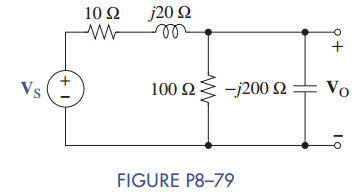Create an Account

Home / Questions / Find the phasor input V S in Figure P8–79 when the phasor output is V O = 300 + j200 V.

Find the phasor input V S in Figure P8–79 when the phasor output is V O = 300 + j200 V.

Find the phasor input VS in Figure P8–79 when the phasor output is VO = 300 + j200 V.Jul 27 2020 View more View LessSubscribe To Get Solution